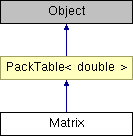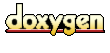Main Page   Namespace List   Class Hierarchy   Compound List   File List   Namespace Members   Compound Members   File Members

# Matrix Class Reference

Mathematical matrix. More...

`#include <mmatrix.h>`

Inheritance diagram for Matrix:List of all members.

## Public Methods

void make (int rs, int cs)
const Matrix & multiplyToSum (double s)
double det () const
void splitVertical (Matrix &a, Matrix &b, int column) const
void splitHorizontal (Matrix &a, Matrix &b, int column) const
Matrix sub (int row0, int row1, int col0, int col1) const
void mulRowByScalar (int row, double scalar)
void addRowByScalar (int srcrow, int dstrow, double scalar)
void swaprows (int row1, int row2)
Matrix complement (int row, int col) const
void joinVertical (const Matrix &a, const Vector &b)
void joinVertical (const Matrix &a, const Matrix &b)
void joinHorizontal (const Matrix &a, const Matrix &b)
const Matrix & operator= (double x)
const Matrix & operator+= (const Matrix &other)
const Matrix & operator+= (double k)
const Matrix & operator *= (const Matrix &other)
const Matrix & operator *= (double k)
const Matrix & operator= (const Matrix &other)

## Detailed Description

Mathematical matrix.

Definition at line 40 of file mmatrix.h.

## Member Function Documentation

 void addRowByScalar ( int srcrow, int dstrow, double scalar )
 Multiplies a row by a scalar and adds it to another row. Rounds values to zero if they are below threshold ROUND_TO_ZERO_THRESHOLD. Definition at line 226 of file mmatrix.cc. References PackTable< double >::cols, and PackTable< double >::get(). Referenced by det(), and solveLinear().

 Matrix complement ( int row, int col ) const
 Forms the complement of the matrix by the given element. Definition at line 197 of file mmatrix.cc. Referenced by det().

 double det ( ) const
 Computes the determinant of the matrix. Definition at line 160 of file mmatrix.cc.

 void joinHorizontal ( const Matrix & a, const Matrix & b )
 Appends two matrices horizontally. The number of columns in both matrices must be equal. Definition at line 392 of file mmatrix.cc.

 void joinVertical ( const Matrix & a, const Matrix & b )
 Appends two matrices vertically. The number of rows in both matrices must be equal. Definition at line 377 of file mmatrix.cc.

 void joinVertical ( const Matrix & a, const Vector & b )
 Appends a vertical vector to matrix. The vector must have length equal to number of rows in the matrix. Definition at line 356 of file mmatrix.cc. Referenced by solveLinear().

 void load ( FILE * in )
 Load matrix from stream. Columns have to be whitespace-separated, rows newline-separated. Definition at line 78 of file mmatrix.cc. References List::add(), String::chop(), PackTable< double >::cols, PackTable< double >::get(), String::length(), make(), String::reserve(), PackTable< double >::rows, and String::toDouble().

 void load ( const String & filename )
 Load matrix from file. Columns have to be whitespace-separated, rows newline-separated. Definition at line 65 of file mmatrix.cc.

 void make ( int rs, int cs ) ` [virtual]`

Creates or recreates the Table with the given dimensions.

If the Table has any old contents, they are destroyed.

Parameters:
 nrows Number of rows in the Table. ncols Number of columns in the Table.

Reimplemented from PackTable< double >.

Definition at line 55 of file mmatrix.cc.

References PackTable::make(), and operator=().

Referenced by joinHorizontal(), joinVertical(), load(), operator=(), splitHorizontal(), splitVertical(), and sub().

 void mulRowByScalar ( int row, double scalar )
 Multiplies a row by a scalar. Definition at line 216 of file mmatrix.cc. References PackTable< double >::cols, and PackTable< double >::get(). Referenced by solveLinear().

 const Matrix & multiplyToSum ( double s )
 Makes the sum of the matrix a given value. Definition at line 138 of file mmatrix.cc. References operator *=(), and sum().

 const Matrix & operator *= ( double x )
 Scales the elements in the matrix with the given value. Definition at line 296 of file mmatrix.cc. References PackTable< double >::cols, and PackTable< double >::rows.

 const Matrix & operator *= ( const Matrix & other )
 Element-by-element multiplication by another matrix. Definition at line 287 of file mmatrix.cc. Referenced by multiplyToSum().

 const Matrix & operator+= ( double x )
 Adds a value to all the elements in the matrix. Definition at line 278 of file mmatrix.cc. References PackTable< double >::cols, and PackTable< double >::rows.

 const Matrix & operator+= ( const Matrix & other )
 Adds the other matrix to the matrix. Definition at line 269 of file mmatrix.cc.

 const Matrix & operator= ( const Matrix & orig )
 Matrix copy operation. Definition at line 259 of file mmatrix.cc.

 const Matrix & operator= ( double x )
 Sets the entire matrix to the given value. Definition at line 250 of file mmatrix.cc. References PackTable< double >::cols, and PackTable< double >::rows. Referenced by make().

 void splitHorizontal ( Matrix & a, Matrix & b, int row ) const
 Splits the matrix row-wise to the given two matrices. Definition at line 341 of file mmatrix.cc.

 void splitVertical ( Matrix & a, Matrix & b, int column ) const
 Splits the matrix column-wise to the given two matrices. Definition at line 328 of file mmatrix.cc.

 Matrix sub ( int row0, int row1, int col0, int col1 ) const
 Returns submatrix. Definition at line 405 of file mmatrix.cc.

 void swaprows ( int row1, int row2 )
 Exchanges the values in two rows. Definition at line 239 of file mmatrix.cc. References PackTable< double >::cols. Referenced by solveLinear().

The documentation for this class was generated from the following files:
Generated on Thu Feb 10 20:06:43 2005 for LibMagiC by1.2.18